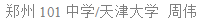# 状态压缩

## Content

### Introduction

• 基本运算符
 名称 C/C++样式 Pascal样式 简记法则 按位与 (bitwise AND) & and 全一则一 否则为零 按位或 (bitwise OR) | or 有一则一 否则为零 按位取反 (bitwise NOT) ~ not 是零则一 是一则零 按位异或 (bitwise XOR) ^ xor 不同则一 相同则零

• 特殊应用
1. and：
1. 用以取出一个数的某些二进制位
2. 取出一个数的最后一个1(lowbit) ：x&-x
2. or ：用以将一个数的某些位设为1
3. not：用以间接构造一些数：~0=4294967295=232-1
4. xor：
1. 不使用中间变量交换两个数：a=a^b;b=a^b;a=a^b;
2. 将一个数的某些位取反

### Getting Started

【引例】

【分析】

①前两行在第3、4列放置了棋子(不考虑顺序，下同)，第三行在第1列放置；②前两行在第1、4列放置了棋子，第三行在第3列放置；

③前两行在第1、3列放置了棋子，第三行在第4列放置。

f=f+f+f

f=1

f[s]=∑f[s^2i]

### Sample Problems

【例1】

【分析】

【例2】

【分析】

f=1;

f[i][j][k]=∑f[i-1][p][k-c[j]]

【例3】

【分析】

f=1;

f[i][j][k]=∑f[i-1][p][k-c[j]]

【例4】

【分析】

f[i][j][k]=max{f[i-1][k][l]}+c[j]

【例5】

【分析】

f[1…11]=1

f[i][s1]=∑f[i-1][s2]

DFS共5个参数，分别为：p(当前列号)，s1、s2(当前行和上一行的覆盖情况)，b1、b2(上一列的放置对当前列上下两行的影响，影响为1否则为0)。初始时s1=s2=b1=b2=0。①p=p+1，s1=s1*2+1，s2=s2*2，b1=b2=0；②p=p+1，s1=s1*2+1，s2=s2*2+1，b1=1，b2=0；③p=p+1，s1=s1*2，s2=s2*2+1，b1=b2=0。当p移出边界且b1=b2=0时记录此方案。

DFS过程有了，实现方法却还有值得讨论的地方。前面的例题中，我们为什么总是把放置方案DFS预处理保存起来？是因为不合法的状态太多，每次都重新DFS太浪费时间。然而回到这个题目，特别是当采用第二种时，我们的DFS过程中甚至只有一个判断(递归边界)，说明根本没有多少不合法的方案，也就没有必要把所有方案保存下来，对于每行都重新DFS即可，这不会增加运行时间却可以节省一些内存。

h=1,h=2

h[i]=2*h[i-1]+h[i-2]

【例6】

【分析】

f[1…11]=1

f[i][s1]=∑f[i-1][s2]

 覆盖情况 条件 参数s变化 参数b变化 1 0 0 0 0 无 s1=s1*2+b1 s2=s2*2+1-b2 b1=0 b2=0 2 0 0 1 1 b1=0 s1=s1*2+1 s2=s2*2+1-b2 b1=1 b2=0 3 1 0 1 0 b1=0 b2=0 s1=s1*2+1 s2=s2*2 b1=0 b2=0 4 1 0 1 1 b1=0 b2=0 s1=s1*2+1 s2=s2*2 b1=1 b2=0 5 0 1 1 1 b1=0 s1=s1*2+1 s2=s2*2+1-b2 b1=1 b2=1 6 1 1 0 1 b2=0 s1=s1*2+b1 s2=s2*2 b1=1 b2=1 7 1 1 1 0 b1=0 b2=0 s1=s1*2+1 s2=s2*2 b1=0 b2=1

【例7】

【分析】

h[i]=ri (i=0~r-1)

h[j]=r*h[j-1]+h[j-r] (j=r~m)04-251754
10-16194802-043万+
07-161万+
03-06
11-26
08-1845